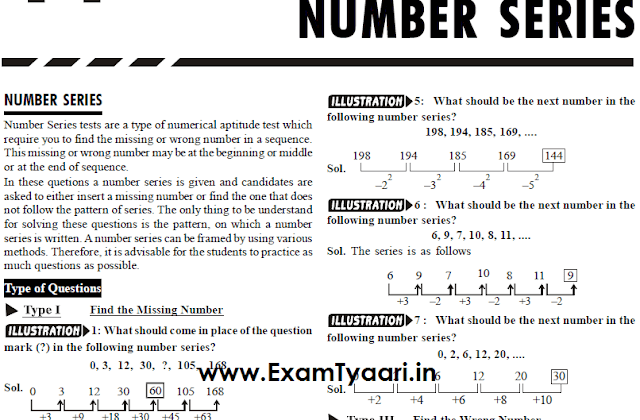# ALGEBRA Formulas Notes & Shortcuts for Competitive Exams [PDF]

### Advance Handwritten Algebra Math Formulas Notes and Shortcuts for SSC & IBPS Exams – PDF Download

Hi Friends,
Algebra questions in the questions papers of competitive exams are very important to score high marks. Algebra is the complete mathematics of formulas. ALgebra formulas are enough to solve any algebraic equation or questions if approached right way.
In this post we have shared a high quality all in one pdf for all the algebra related concept notes, shortcuts and formulas with solved examples. Download this pdf and remember all the important algebra formulas. Solved examples using all the mathematical identities and formulas are also included in the pdf.

This Handwritten Notes PDF includes below…

• Study Notes
• Concept Notes
• Formulas
• Solved Examples
• Hindi Notes

Download the PDF for all the competitive exams like ssc cgl, chsl, cpo and other ibps bank po and clerk exams.

DETAILS :
Credits: Mayank Verma
QUality: HIGH Quality
Size: 26 MB
Pages: 148